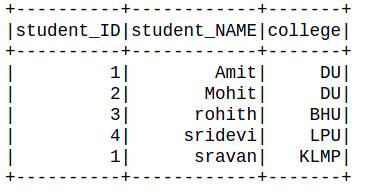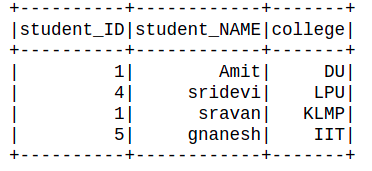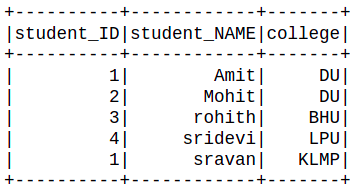Open in App
Not now

# Delete rows in PySpark dataframe based on multiple conditions

• Last Updated : 29 Jun, 2021

In this article, we are going to see how to delete rows in PySpark dataframe based on multiple conditions.

### Method 1: Using Logical expression

Here we are going to use the logical expression to filter the row. Filter() function is used to filter the rows from RDD/DataFrame based on the given condition or SQL expression.

Syntax: filter( condition)

Parameters:

• Condition: Logical condition or SQL expression

Example 1:

## Python3

 `# importing module``import` `pyspark`` ` `# importing sparksession from pyspark.sql``# module``from` `pyspark.sql ``import` `SparkSession`` ` `# spark library import``import` `pyspark.sql.functions`` ` `# creating sparksession and giving an app name``spark ``=` `SparkSession.builder.appName(``'sparkdf'``).getOrCreate()`` ` `# list  of students  data``data ``=` `[[``"1"``, ``"Amit"``, ``" DU"``],``        ``[``"2"``, ``"Mohit"``, ``"DU"``],``        ``[``"3"``, ``"rohith"``, ``"BHU"``],``        ``[``"4"``, ``"sridevi"``, ``"LPU"``],``        ``[``"1"``, ``"sravan"``, ``"KLMP"``],``        ``[``"5"``, ``"gnanesh"``, ``"IIT"``]]`` ` `# specify column names``columns ``=` `[``'student_ID'``, ``'student_NAME'``, ``'college'``]`` ` `# creating a dataframe from the lists of data``dataframe ``=` `spark.createDataFrame(data, columns)`` ` `dataframe ``=` `dataframe.``filter``(dataframe.college !``=` `"IIT"``)`` ` `dataframe.show()`

Output:Example 2:

## Python3

 `# importing module``import` `pyspark`` ` `# importing sparksession from pyspark.sql``# module``from` `pyspark.sql ``import` `SparkSession`` ` `# spark library import``import` `pyspark.sql.functions`` ` `# creating sparksession and giving an app name``spark ``=` `SparkSession.builder.appName(``'sparkdf'``).getOrCreate()`` ` `# list  of students  data``data ``=` `[[``"1"``, ``"Amit"``, ``" DU"``],``        ``[``"2"``, ``"Mohit"``, ``"DU"``],``        ``[``"3"``, ``"rohith"``, ``"BHU"``],``        ``[``"4"``, ``"sridevi"``, ``"LPU"``],``        ``[``"1"``, ``"sravan"``, ``"KLMP"``],``        ``[``"5"``, ``"gnanesh"``, ``"IIT"``]]`` ` `# specify column names``columns ``=` `[``'student_ID'``, ``'student_NAME'``, ``'college'``]`` ` `# creating a dataframe from the lists of data``dataframe ``=` `spark.createDataFrame(data, columns)`` ` `dataframe ``=` `dataframe.``filter``(``    ``((dataframe.college !``=` `"DU"``)``     ``& (dataframe.student_ID !``=` `"3"``))``)`` ` `dataframe.show()`

Output:### Method 2: Using when() method

It evaluates a list of conditions and returns a single value. Thus passing the condition and its required values will get the job done.

Syntax: When( Condition, Value)

Parameters:

• Condition: Boolean or columns expression.
• Value: Literal Value

Example:

## Python3

 `# importing module``import` `pyspark`` ` `# importing sparksession from pyspark.sql ``# module``from` `pyspark.sql ``import` `SparkSession`` ` `# spark library import``import` `pyspark.sql.functions`` ` `# spark library import``from` `pyspark.sql.functions ``import` `when`` ` `# creating sparksession and giving an app name``spark ``=` `SparkSession.builder.appName(``'sparkdf'``).getOrCreate()`` ` `# list  of students  data``data ``=` `[[``"1"``, ``"Amit"``, ``" DU"``],``        ``[``"2"``, ``"Mohit"``, ``"DU"``],``        ``[``"3"``, ``"rohith"``, ``"BHU"``],``        ``[``"4"``, ``"sridevi"``, ``"LPU"``],``        ``[``"1"``, ``"sravan"``, ``"KLMP"``],``        ``[``"5"``, ``"gnanesh"``, ``"IIT"``]]`` ` `# specify column names``columns ``=` `[``'student_ID'``, ``'student_NAME'``, ``'college'``]`` ` `# creating a dataframe from the lists of data``dataframe ``=` `spark.createDataFrame(data, columns)`` ` `dataframe.withColumn(``'New_col'``,``                     ``when(dataframe.student_ID !``=` `'5'``, ``"True"``)``                     ``.when(dataframe.student_NAME !``=` `'gnanesh'``, ``"True"``)``                     ``).``filter``(``"New_col == True"``).drop(``"New_col"``).show()`

Output:My Personal Notes arrow_drop_up